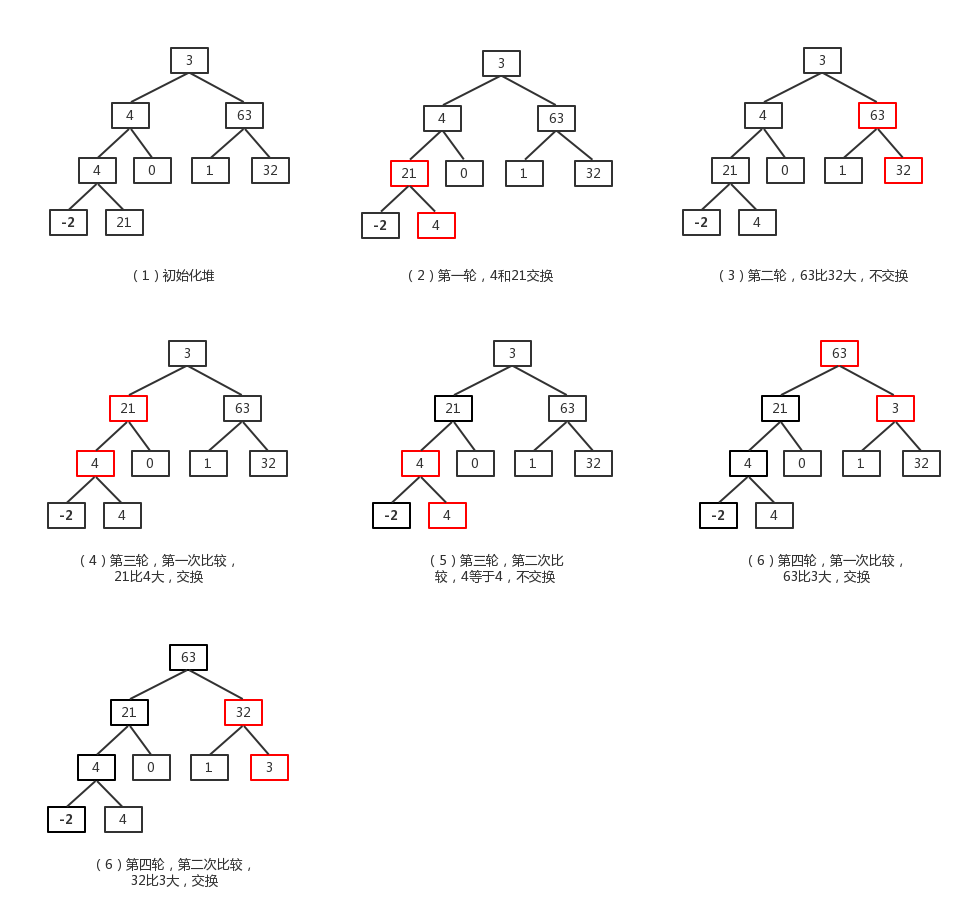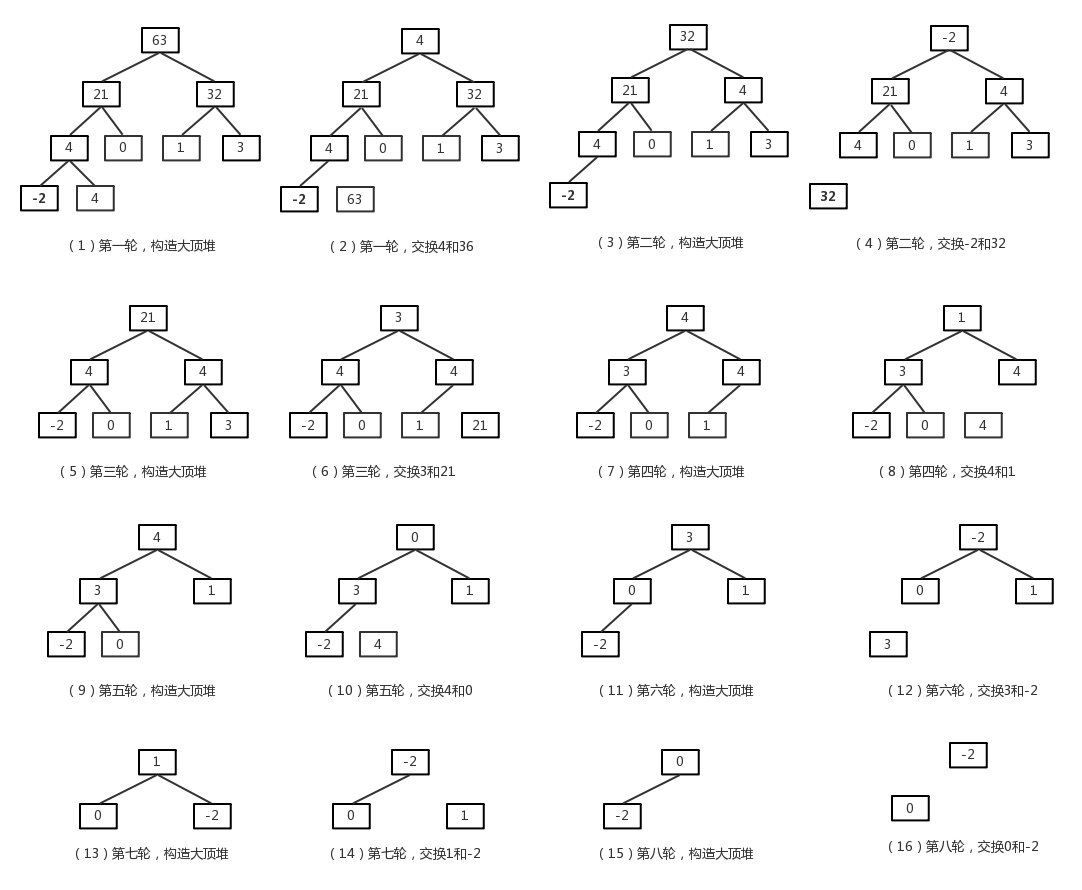# 基础概念

## 堆

• 堆是一棵完全二叉树。
• 堆中每个结点的值总是不大于（或不小于）其子结点的值。

• 它的左孩子结点为：R[2*i+1]。
• 它的右孩子结点为：R[2*i+2]。
• 它的父结点为：R[(i-1)/2]。
• R[i]>=R[2*i+1] 且 R[i]>=R[2*i+2]

# 排序思想

1. 将数组构造成一个大顶堆。
2. 交换第一个（堆的根节点）和最后一个元素（堆上最后一层最右的叶子结点）即把大的元素放到数组最后。然后在将去除最后一个元素的数组重新构造大顶堆。

# 构造大顶堆

## 构造步骤

1. 取R[i]的子结点中较大的结点R[2*i+1]（此处假设左结点大于右结点）。
2. 若R[2*i+1]不大于R[i]，则结束。否则，交换R[i]和R[2*i+1]，交换完成后，以R[2*i+1]为根结点，重复上述步骤。

## 构造示例# 堆排序过程# 详细代码

public int[] sort(int[] array) {
int length = array.length;
while (length > 1) {
initHeap(array, length);

System.out.println("初始化堆结果：" + Arrays.toString(array));

int temp = array[length - 1];
array[length - 1] = array;
array = temp;

System.out.println("排序结果：" + Arrays.toString(array));
length--;
}
return array;
}

private void initHeap(int[] array, int length) {
// 从最后一个非叶子结点的父结点开始
for (int i = (length - 2) / 2; i >= 0; i--) {
int parent = i;
int parentValue = array[parent]; // 记录下parent的值
int maxChild = 2 * parent + 1; // 先选取左结点为大结点

while (maxChild < length) {
// 如果有右结点且右结点大于左结点，选取右结点为大结点
if ((maxChild + 1) < length && array[maxChild] < array[maxChild + 1]) {
maxChild++;
}

// 如果大结点小于父结点直接结束
if (array[maxChild] <= parentValue) {
break;
}

// 将大结点的值赋值给父结点
array[parent] = array[maxChild];
// 发生交换后，继续调整已大结点为根结点的树
parent = maxChild;
maxChild = 2 * parent + 1;
}

array[parent] = parentValue;
}
}

# 优化后代码

private static void adjustHeat(int[] array, int parent, int length) {
// parentValue保存当前父节点
int parentValue = array[parent];
// 先选取左结点为大结点
int maxChild = 2 * parent + 1;

while (maxChild < length) {
// 如果有右结点且右结点大于左结点，选取右结点为大结点
if ((maxChild + 1) < length && array[maxChild] < array[maxChild + 1]) {
maxChild++;
}

// 如果大结点小于父结点直接结束
if (array[maxChild] <= parentValue) {
break;
}

// 将大结点的值赋值给父结点
array[parent] = array[maxChild];
// 发生交换后，继续调整以大结点为根结点的树
parent = maxChild;
maxChild = 2 * parent + 1;
}
array[parent] = parentValue;
}

public void sort(int[] array) {
// 循环建立初始堆
for (int i = (array.length - 2) / 2; i >= 0; i--) {
}

// 进行n-1次循环，完成排序
for (int length = array.length - 1; length > 0; length--) {
// 最后一个元素和第一元素进行交换
int temp = array[length];
array[length] = array;
array = temp;

System.out.println(String.format("第 %d 趟排序结果：", array.length - length) + Arrays.toString(array));

// 将剩下数组总元素组成的堆继续调整
}